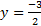# Solving Equations with Variables on Both Sides

 Sometimes an equation will have a variable on both sides. What do you think you should do with an equation like this? 5x+2=3x-1 If you're thinking that you would add 5x and 3x, you are missing something very important: These terms are on opposite sides of the equation and therefore cannot be added. You can only add terms that are on the same side. Because we can only add terms when they are on the same side, we have to move one of the terms to the other side by adding its opposite to both sides. Here's how this works: 5x+2=3x-1 5x-3x+2=3x-3x-1 5x-3x+2=-1 As you can see, we moved the 3x to the left hand side by adding its opposite to both sides. The opposite of 3x is -3x. The 3x and -3x combine to equal 0, thus disappearing from the right side. We now have an equation like the ones we solved previously and we can continue as normal. The next step is to add like terms, which we are now able to do since they are on the same side. Let's finish the problem: 5x-3x+2=-1 2x+2=-1 2x+2-2=-1-2 2x=-3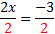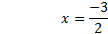Let's look at one more example: 3y+8=-6y-10 3y+6y+8=-6y+6y-10 3y+6y+8=-10 9y+8=-10 9y+8-8=-10-8 9y=-18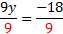y=-2 Practice:Solve the following equations: 1) 8y+2=10y+3 2) -6x+1=3x-2 3) 10x-2=x+7 4) 5a+9=-2a+11 5) 3y+3y-2=8y+1 Answers: 1)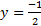2)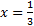3) x=1 4) a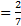5)